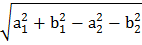# Ifandare three given points, then locus of the pointsatisfying the relationis a) A straight line parallel to-axis b) A circle passing through the origin c) A circle with the centre at the origin d) A straight line parallel to-axis

## Question ID - 53551 :- Ifandare three given points, then locus of the pointsatisfying the relationis a) A straight line parallel to-axis b) A circle passing through the origin c) A circle with the centre at the origin d) A straight line parallel to-axis

3537

(d)

We have. Let. Now given that. Hence,Which is a straight line parallel to-axis

Next Question :
 If the equation of the locus of a point equidistant  from the pointsandis, then the value ofis a)b)c)d)